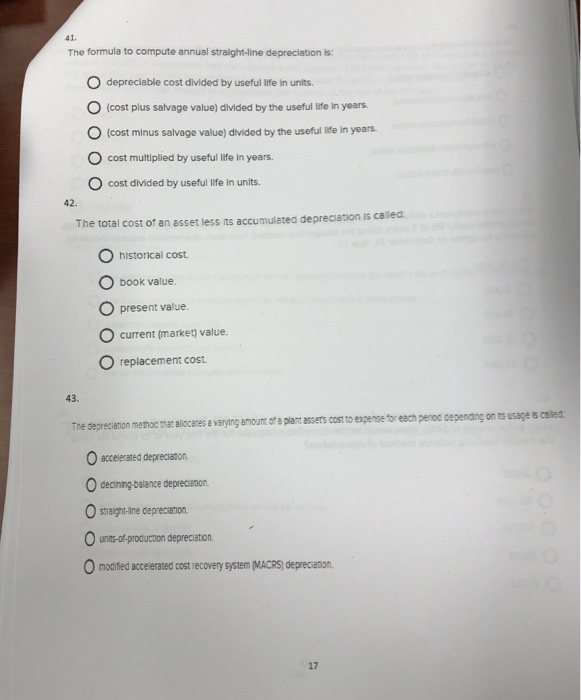# The formula to compute annual straight line depreciation is41. The formula to compute annual straight-line depreclation is: O depreciable cost divided by useful life in units (cost plus salvage value) divided by the useful life in years. O (cost minus salvage value) divided by the useful life in years cost multiplied by useful life in years O cost divided by useful life In units. 42. The total cost of an asset less its accumulated depreciation is called O historical cost. O book value. O present value O current (markeq value. replacement cost 43. The depreciaton memoc tmar alocanes e varying amount of e plamassers cost to expense for eech penoc cepending on is ussge is caliled O accelerated depreciason O dectinng-balance deprecanon. O straigh -ane deprecanion. O modifed accelerated cost recovery system MACRS) deprecaton 17

Answer:-41)-The formula to compute annual straight line
depreciation is:- (cost minus salvage value) divided by the useful
life in years.
Explanation:- Straight line Method:-
= Cost of asset- Salvage value of asset/No. of useful life
(years)
42)-The total cost of an asset less an accumulated depreciation
is called:-Book value
43)-The depreciation method allocates a varying amount of a
plant assets cost to expense for each period depending on its usage
is called:-Unit-of-production depreciation.
Explanation:- Units of production method:-
Annual depreciation expense per unit produced=Cost – salvage
/Total units produced